Question

In a Titration 50.00mL of LiOH was titrated with a 0.1000M HBr solution. At the endpoint, the volume of acid (the titrant) used was 37.87 mL. What was the concentration of the base before the titration began? (What is the concentration of LiOH in the original solution of LiOH)? show all work.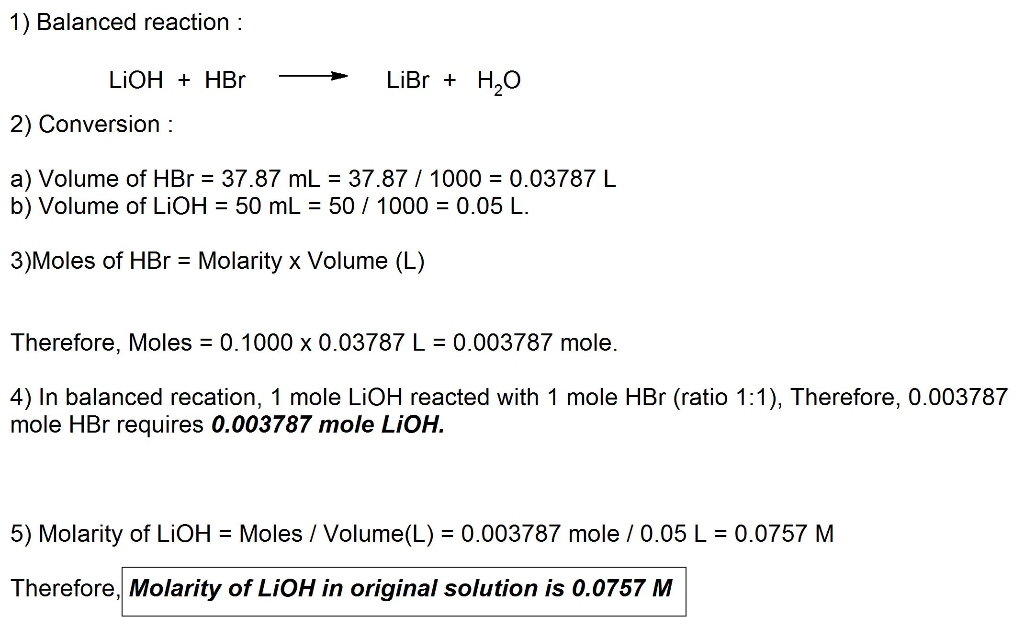#### Earn Coins

Coins can be redeemed for fabulous gifts.

Similar Homework Help Questions
• ### Calculate the pH at the titration endpoint, when 35.00 mL of 0.150 M dimethylamine is titrated...

Calculate the pH at the titration endpoint, when 35.00 mL of 0.150 M dimethylamine is titrated by 0.170 M hydrochloric acid. Please show all work

• ### 1) A 25.00 mL sample of HBr is titrated with a 0.150 M standardized sodium hydroxide...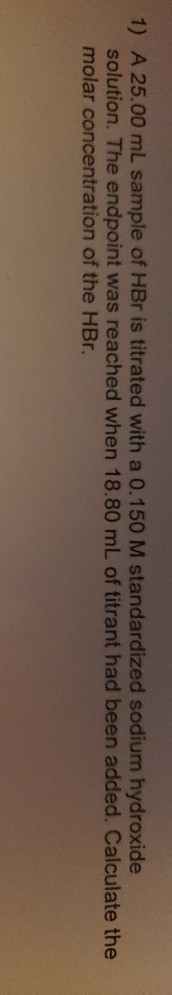1) A 25.00 mL sample of HBr is titrated with a 0.150 M standardized sodium hydroxide solution. The endpoint was reached when 18.80 mL of titrant had been added. Calculate the molar concentration of the HBr.

• ### Titration of a Weak Base Prelaboratory Questions 1. Will addition of HCl to a solution make...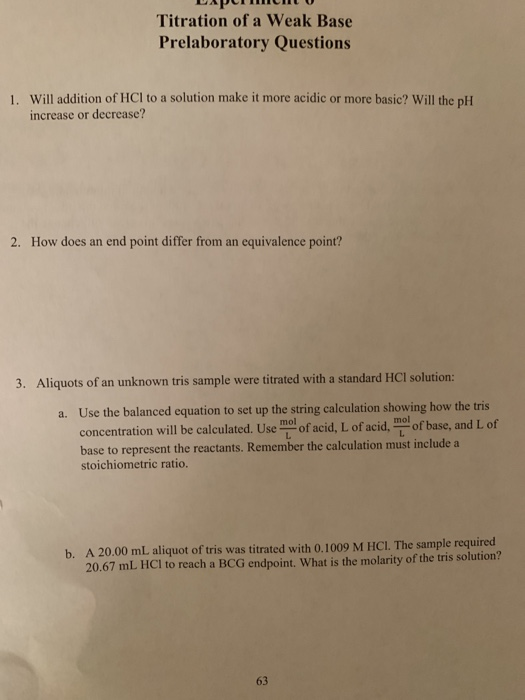Titration of a Weak Base Prelaboratory Questions 1. Will addition of HCl to a solution make it more acidic or more basic? Will the pH increase or decrease? How does an end point differ from an equivalence point? 2. were titrated with a standard HCl solution: 3. Aliquots of an unknown tris sample Use the balanced equation to set up the string calculation showing how the tris a. mol of acid, L of acid. mo of base, and L of...

• ### A 25.00 mL sample of NaOH was titrated with a 0.743 M H2sO, solution. The endpoint of the titration was observed aft...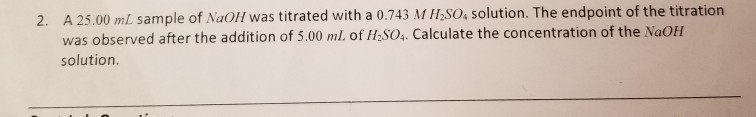A 25.00 mL sample of NaOH was titrated with a 0.743 M H2sO, solution. The endpoint of the titration was observed after the addition of 5.00 ml. of H2SO4. Calculate the concentration of the NaOH 2. solution.

• ### True or False: During a titration the solution of UNKNOWN concentration should be placed in the...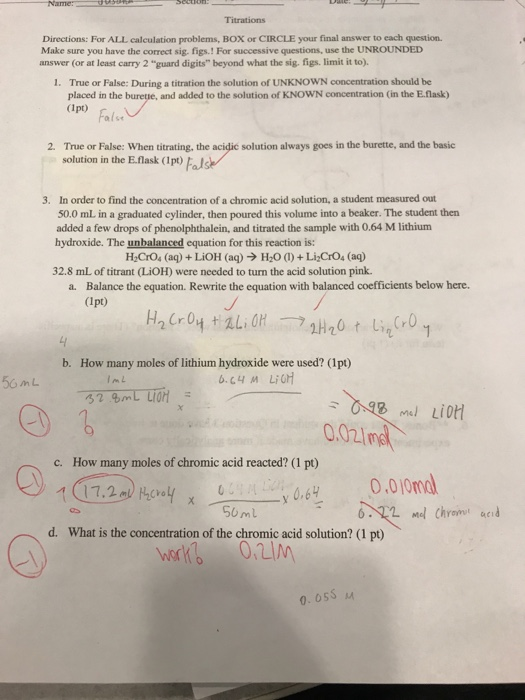True or False: During a titration the solution of UNKNOWN concentration should be placed in the burette, and added to the solution of KNOWN concentration (in the E.flask) True or False: When titrating, the acidic solution always goes in the burette, and the basic solution in the E.flask) In order to find the concentration of a chromic acid solution, a student measured out 50.0 mL in a graduated cylinder, then poured this volume into a beaker. The student then added...

• ### The titration curve shown below represents a 25 mL aqueous solution that is titrated with another...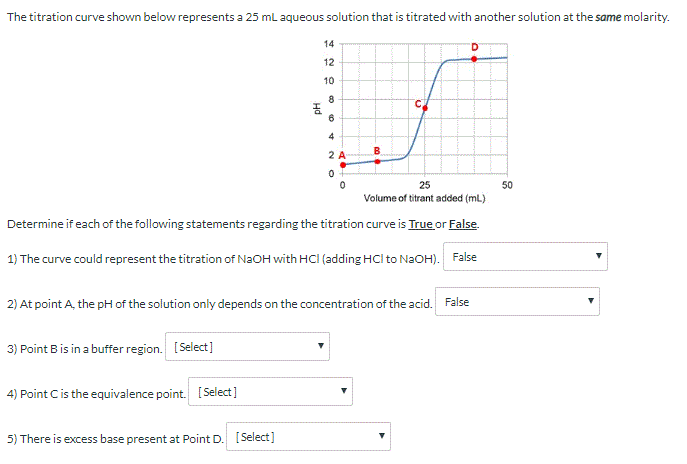The titration curve shown below represents a 25 mL aqueous solution that is titrated with another solution at the same molarity. PH 50 25 Volume of titrant added (ml) Determine if each of the following statements regarding the titration curve is True or False. 1) The curve could represent the titration of NaOH with HCl (adding HCl to NaOH). False 2) At point A, the pH of the solution only depends on the concentration of the acid. False 3) Point...

• ### A vinegar (acetic acid) solution of unknown concentration was titrated to the light pink endpoint with the standardized NaOH solution.

A vinegar (acetic acid) solution of unknown concentration was titrated to the light pink endpoint with the standardized NaOH solution. The weight volume % of the vinegar solution were calculated. Molecular formula of Acetic acid: C2H4O2 Volume of vinegar sample titrated (ml) 5.00 Volume of NaOH required to neutralize vinegar in (mL) 8.74 Concentration of NaOH in mol/L, 0.1979 Calculate the weight/volume percentage of the vinegar solution (g/100 ml).

• ### It's a weak acid strong base titration Experiment 4: Identification of an unknown acid by titration...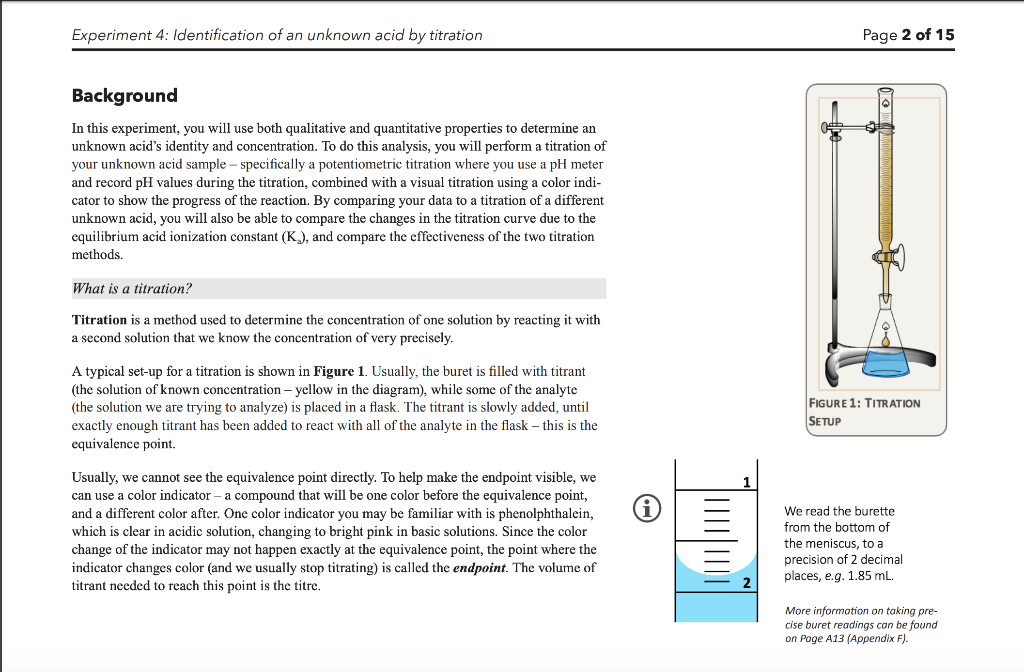It's a weak acid strong base titration Experiment 4: Identification of an unknown acid by titration Page 2 of 15 Background In this experiment, you will use both qualitative and quantitative properties to determine an unknown acid's identity and concentration. To do this analysis, you will perform a titration of your unknown acid sample-specifically a potentiometric titration where you use a pH meter and record pH values during the titration, combined with a visual titration using a color indi- cator...

• ### Experiment 13 Acid-Base Equilibria, Part I1 Lab partner Report Sheet Data Titration 1: Solution being titrated...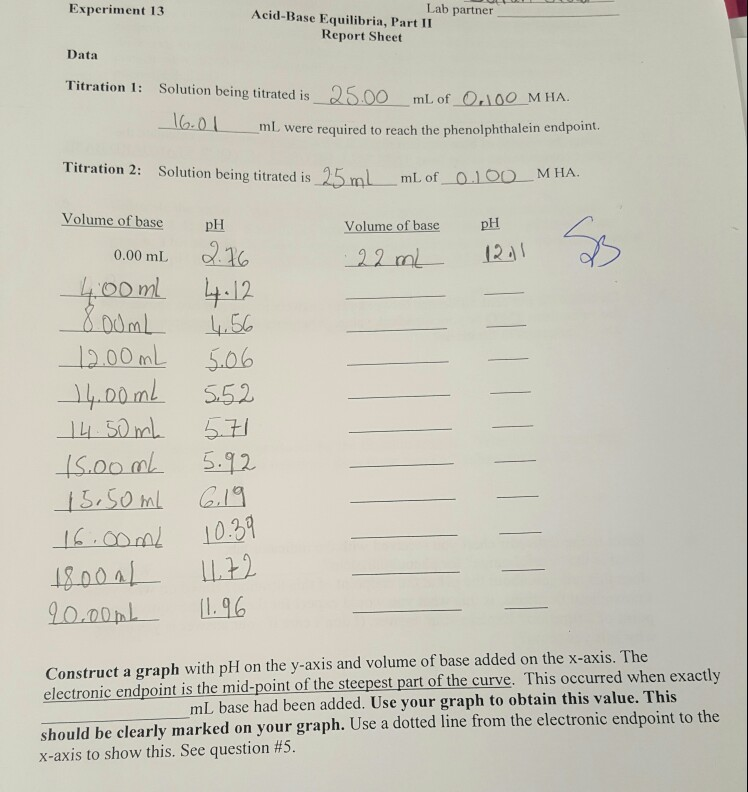Experiment 13 Acid-Base Equilibria, Part I1 Lab partner Report Sheet Data Titration 1: Solution being titrated is Q500 ml of 100 MHA. mI, were required to reach the phenolphthalein endpoint Titration 2: Solution being titraed is 25ml ml of MHA Volume of base p pH Volume of base 0.00 mL 05.0% 5.52 ISoom 592 L.96 Construct a graph with pH on the y-axis and volume of base added on the x-axs. The electronic endpoint is the mid-point of the steepest...

• ### 1. What is the definition of an 'equivalence point' in an acid/base titration? (1 point) 2....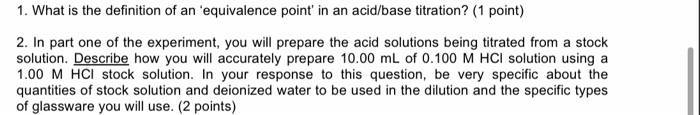1. What is the definition of an 'equivalence point' in an acid/base titration? (1 point) 2. In part one of the experiment, you will prepare the acid solutions being titrated from a stock solution. Describe how you will accurately prepare 10.00 mL of 0.100 M HCl solution using a 1.00 M HCl stock solution. In your response to this question, be very specific about the quantities of stock solution and deionized water to be used in the dilution and the...